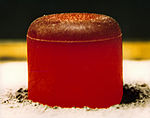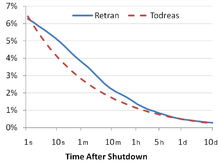# Decay heat

﻿
Decay heatRTG pellet glowing red because of the heat generated by the radioactive decay of plutonium-238 dioxide, after a thermal isolation test.

Decay heat is the heat released as a result of radioactive decay. This is when the radiation interacts with materials: the energy of the alpha, beta or gamma radiation is converted into the thermal movement of atoms.

## Natural occurrence

Naturally occurring decay heat is a significant source of the heat in the interior of the Earth. Radioactive isotopes of uranium, thorium and potassium are the primary contributors to this decay heat.

## Power reactors in shutdownDecay heat as fraction of full power for a reactor SCRAMed from full power at time 0, using two different correlations

In a typical nuclear fission reaction, 187 MeV of energy are released instantaneously in the form of kinetic energy from the fission products, kinetic energy from the fission neutrons, instantaneous gamma rays, or gamma rays from the capture of neutrons. An additional 23 MeV of energy are released at some time after fission from the beta decay of fission products. About 10 MeV of the energy released from the beta decay of fission products is in the form of neutrinos, and since neutrinos are very weakly interacting, this 10 MeV of energy will not be deposited in the reactor core. This results in 13 MeV (6.5% of the total fission energy) being deposited in the reactor core after any given fission reaction has occurred.

When a nuclear reactor has been shut down, and nuclear fission is not occurring at a large scale, the major source of heat production will be due to the beta decay of these fission fragments. For this reason, at the moment of reactor shutdown, decay heat will be about 6.5% of the previous core power if the reactor has had a long and steady power history. About 1 hour after shutdown, the decay heat will be about 1.5% of the previous core power. After a day, the decay heat falls to 0.4%, and after a week it will be only 0.2%. The decay heat production rate will continue to slowly decrease over time; the decay curve depends upon the proportions of the various fission products in the core and upon their respective half-lives.  An approximation for the decay heat curve valid from 10 seconds to 100 days after shutdown is$\frac{P}{P_0} = 0.066 \left( \left( \tau - \tau_s \right)^{-0.2} - \tau^{-0.2} \right)$

where P is the decay power, P0 is the reactor power before shutdown, τ is the time since reactor start and τs is the time of reactor shutdown measured from the time of startup (in seconds). For an approach with a more direct physical basis, some models use the fundamental concept of radioactive decay. Used nuclear fuel contains a large number of different isotopes that contribute to decay heat, which are all subject to the radioactive decay law, so some models consider decay heat to be a sum of exponential functions with different decay constants and initial contribution to the heat rate. A more accurate model would consider the effects of precursors, since many isotopes follow several steps in their radioactive decay chain, and the decay of daughter products will have a greater effect longer after shutdown.$\frac{P}{P_0} = \sum_{i=1}^{11}P_i e^{-\lambda t}.$

The removal of the decay heat is a significant reactor safety concern, especially shortly after normal shutdown or following a loss-of-coolant accident. Failure to remove decay heat may cause the reactor core temperature to rise to dangerous levels and has caused nuclear accidents, including the nuclear accidents at Three Mile Island and Fukushima I. The heat removal is usually achieved through several redundant and diverse systems, from which heat is removed via heat exchangers. Water is passed through the secondary side of the heat exchanger via the essential service water system which dissipates the heat into the 'ultimate heat sink', often a sea, river or large lake. In locations without a suitable body of water, the heat is dissipated into the air by recirculating the water via a cooling tower. The failure of ESWS circulating pumps was one of the factors that endangered safety during the 1999 Blayais Nuclear Power Plant flood.

## Spent fuel

After one year, typical spent nuclear fuel generates about 10 kW of decay heat per tonne, decreasing to about 1 kW/t after ten years. Hence effective active or passive cooling for spent nuclear fuel is required for a number of years.